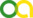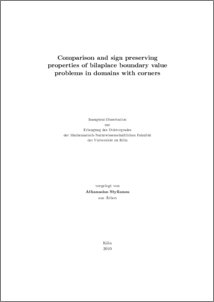Stylianou, Athanasios (2010). Comparison and sign preserving properties of bilaplace boundary value problems in domains with corners. PhD thesis, Universität zu Köln.Preview
PDF
StylianouDissertation.pdf

## Abstract

This work is focused on the study of the Kirchhoff-Love model for thin, transversally loaded plates with corner singularities on the boundary. The former consists in finding a real valued function u, defined in a bounded, planar set. The latter represents the shape of the plate and u(x) its vertical deflection at the point x. This makes sense since we are in the framework of linear elasticity, that is, the model assumes that no horizontal deformation takes place. The function u is found as the minimizer of the Kirchhoff energy functional in different subsets of a suitable Sobolev space, incorporating the boundary conditions. One can distinguish the following cases: (i) clamped: the function u and its exterior normal derivative are assumed to be zero on the boundary of the plate, (ii) hinged: we assume only u=0 on the boundary and (iii) supported: u is assumed nonnegative on the boundary. A hinged plate will additionally satisfy a set of natural boundary conditions, whereas a solution in the supported case will exist only if we assume that the load f pushes the plate down effectively; in that case a set of natural boundary conditions will be again fulfilled. It is however common within the mathematical and engineering literature to confuse the hinged and supported plates. This originates from the expectation that when pressed down, a supported plate, like a supported beam, will have a zero deflection on the boundary. Here we prove the contrary: If the domain has a corner, then a hinged plate cannot be in general a minimizer of the energy functional if we allow variations with positive boundary values. Moreover, we illustrate that a hinged plate with a sufficiently smooth boundary satisfies a comparison principle. In the last chapter we consider the problem of decoupling a clamped plate into a system of second order equations. This approach is very important for numerical procedures, since one can then use standard piecewise linear elements. We show that such a decomposition yields the correct solutions only if the domain has convex corners; when a concave corner is present then the system has no solution.

Item Type: Thesis (PhD thesis)
Creators:
CreatorsEmailORCIDORCID Put Code
Stylianou, Athanasiosastylian@math.uni-koeln.deUNSPECIFIEDUNSPECIFIED
URN: urn:nbn:de:hbz:38-33198
Date: 2010
Language: English
Faculty: Faculty of Mathematics and Natural Sciences
Divisions: Faculty of Mathematics and Natural Sciences > Department of Mathematics and Computer Science > Mathematical Institute
Subjects: Mathematics
Uncontrolled Keywords:
KeywordsLanguage
biharmonic equation , simply supported plate , mixed methods , nonsmooth domainsEnglish
Date of oral exam: 20 October 2010
Referee:View Item